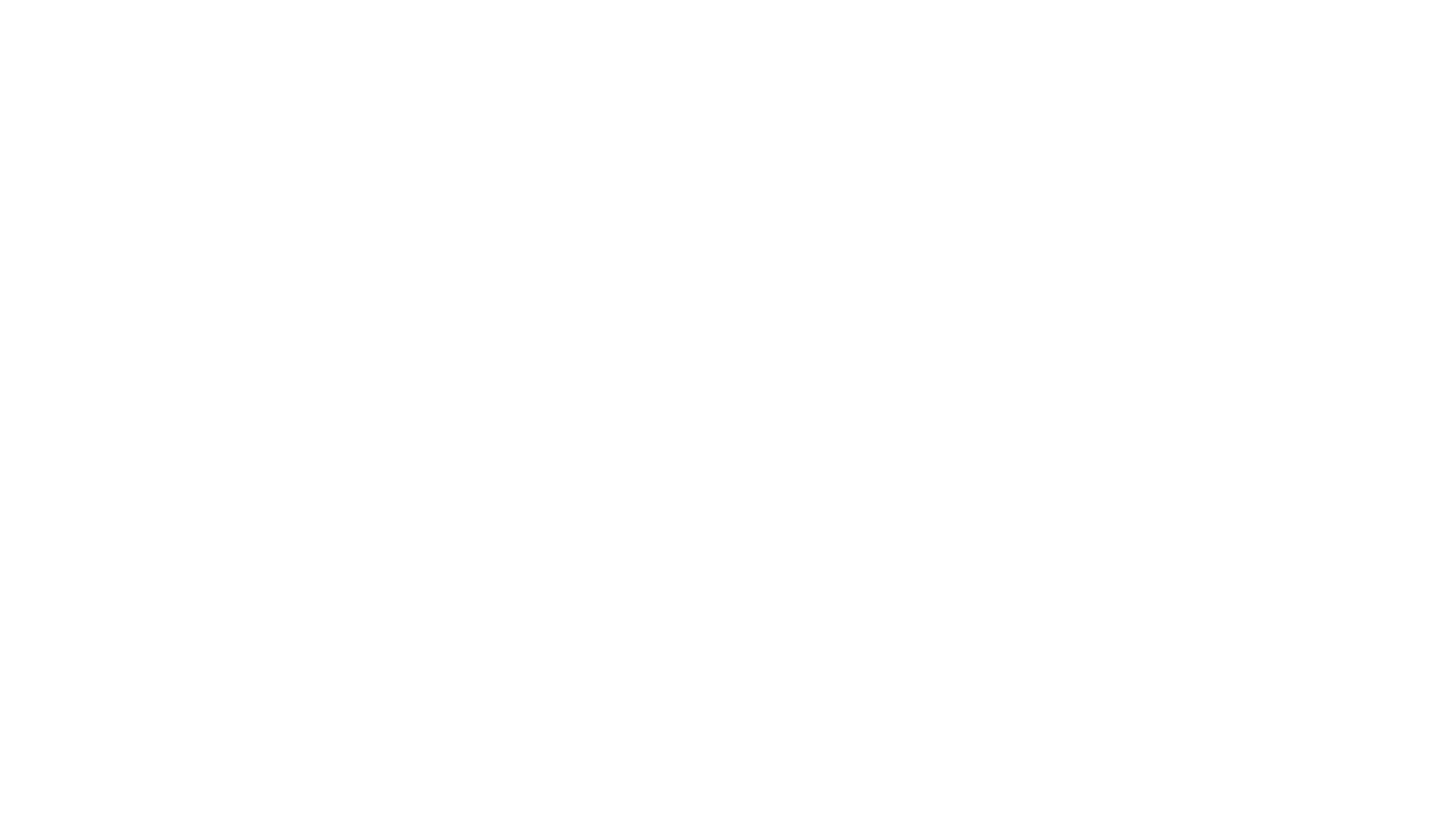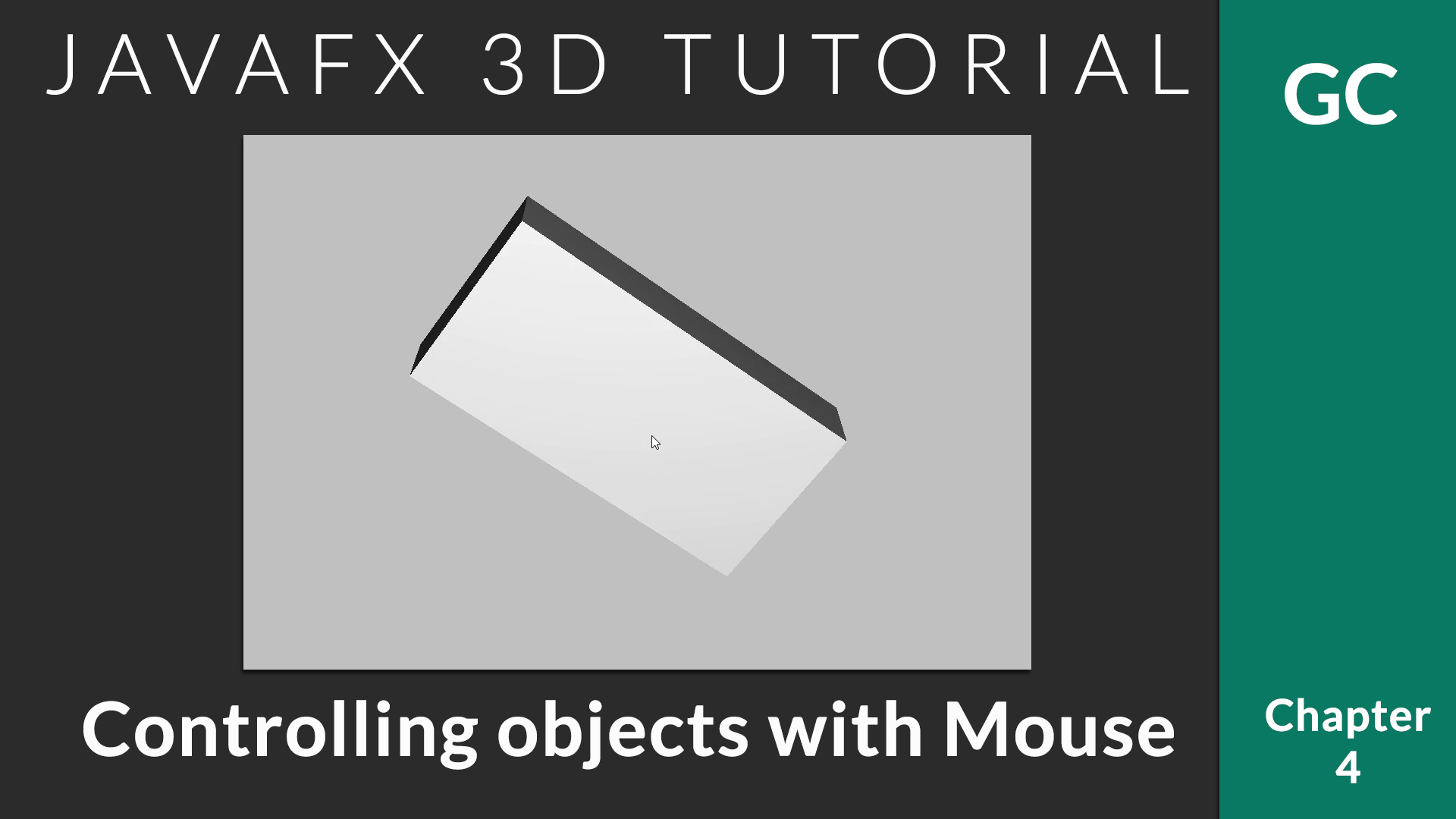Home JavaFX JavaFX 3D JavaFX 3D Tutorial #4 – 3D Object Transform (Rotation) with Mouse

# JavaFX 3D Tutorial #4 – 3D Object Transform (Rotation) with MouseIn the previous chapter, we saw how to do the transforms with keyboard input. In this chapter, we take it a step further. Today, we will talk about JavaFX 3D Mouse Control implementation. Giving control using mouse is more reliable than keyboard. With mouse control, user can directly interact with the 3D application.

The basic idea is to track the mouse drag. Based on how much drag is made in x and y-axis, we apply rotation transformation.

The following method will do the trick.

``` //Tracks drag starting point for x and y
private double anchorX, anchorY;
//Keep track of current angle for x and y
private double anchorAngleX = 0;
private double anchorAngleY = 0;
//We will update these after drag. Using JavaFX property to bind with object
private final DoubleProperty angleX = new SimpleDoubleProperty(0);
private final DoubleProperty angleY = new SimpleDoubleProperty(0);

private void initMouseControl(SmartGroup group, Scene scene) {
Rotate xRotate;
Rotate yRotate;
xRotate = new Rotate(0, Rotate.X_AXIS),
yRotate = new Rotate(0, Rotate.Y_AXIS)
);
xRotate.angleProperty().bind(angleX);
yRotate.angleProperty().bind(angleY);

scene.setOnMousePressed(event -> {
anchorX = event.getSceneX();
anchorY = event.getSceneY();
anchorAngleX = angleX.get();
anchorAngleY = angleY.get();
});

scene.setOnMouseDragged(event -> {
angleX.set(anchorAngleX - (anchorY - event.getSceneY()));
angleY.set(anchorAngleY + anchorX - event.getSceneX());
});
}
```

Let’s discuss the code parts.

##### Step 1: Prepare and apply initial rotation transformation
```//Prepare X and Y axis rotation transformation obejcts
Rotate xRotate;
Rotate yRotate;
//Add both transformation to the container
xRotate = new Rotate(0, Rotate.X_AXIS),
yRotate = new Rotate(0, Rotate.Y_AXIS)
);
/*Bind Double property angleX/angleY with corresponding transformation.
When we update angleX / angleY, the transform will also be auto updated.*/
xRotate.angleProperty().bind(angleX);
yRotate.angleProperty().bind(angleY);
```
##### Step 2: Track starting of mouse drag.

It is necessary to track the drag start. Because, we will have to track how much the drag was happened in both axes.

```//Listen for mouse press -- Drag start with a click :-)
scene.setOnMousePressed(event -> {
//Save start points
anchorX = event.getSceneX();
anchorY = event.getSceneY();
//Save current rotation angle
anchorAngleX = angleX.get();
anchorAngleY = angleY.get();
});
```
##### Step 3: Calculate angle of rotation based on current drag point

When the user start dragging, after each pixel (or minor dragging), we will get a callback. We will check current (x,y) value and compare it with previous to calculate the rotation delta.

```//Listen for drag
scene.setOnMouseDragged(event -> {
/*event.getSceneY() gives current Y value. Find how much far away
it is from saved anchorY point.
*/
angleX.set(anchorAngleX - (anchorY - event.getSceneY()));
angleY.set(anchorAngleY + anchorX - event.getSceneX());
});
```

It is important to note that, when the user drags in Y axis, we have to rotate based on X axis and vice versa (Try the opposite and see how much weird it is). The negative symbol in angleX is to set the direction of rotation. You can change the direction by changing the subtraction to addition.

Find Code in GitHub